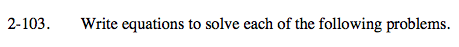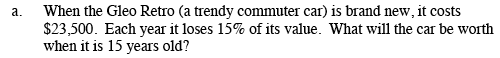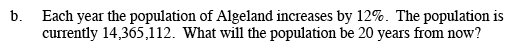Home > A2C > Chapter 2 > Lesson 2.1.7 > Problem2-103

2-103.
1. Write equations to solve each of the following problems. Homework Help ✎

1. When the Gleo Retro (a trendy commuter car) is brand new, it costs $23,500. Each year it loses 15% of its value. What will the car be worth when it is 15 years old? 2. Each year the population of Algeland increases by 12%. The population is currently 14,365,112. What will the population be 20 years from now?You need to know the starting value and multiplier to write the equation. The multiplier for a 15% decrease can be thought of as 100% − 15%. $y=23500\cdot(0.85)^{\textit{x}}$$2052.82$y=14365112\cdot(1.12)^{\textit{x}}$